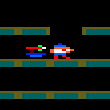### CPC Basic

Would you like to react to this message? Create an account in a few clicks or log in to continue.

# Poking Star LIne Pixels to Screen and moving VerticallyOut Bush

Posts : 11
Join date : 2019-12-02
This is another variation of the Vertical stars I made earlier. Last year I worked out with a series of Loops to determine Blocks and Segments I could fill a whole Screen Line for Line with Random values in Locomotive BASIC. BASIC on that coding I came up with with a way to POKE some pixels (PEN 1 & 2) and have them move across the screen and as I thought the result works a little bit quicker than the earlier version which uses PLOT to do.

Code:
`100 MODE 0:BORDER 0:INK 0,0:INK 1,26:INK 2,13110 DEFBYTE p : DEFWORD a-o,q-z : DIM p(2), x(10), y(10), b(10), s(10), z(10) : p(1)=128 : p(2) = 8120 c = 0130 WHILE c <> 10140  y(c) = RND MOD 7150  x(c) = RND MOD 79160  b(c) = RND MOD 25 : z(c) = b(c) * 80170  s(c) = p(RND MOD 2 + 1)180  GOSUB 1000190  c = c + 1200 WEND210 ' Main Loop220 c = 0230 WHILE 1240  IF x(c) = 0 THEN GOSUB 1100 : GOSUB 2000 : s(c) = p(RND MOD 2 + 1)250  IF x(c) > 0 THEN GOSUB 1100 : x(c) = x(c) - 1260  GOSUB 1000270  c = c + 1280  IF c=10 THEN CALL &BD19 : c=0290 WEND1000 POKE (&C000+(y(c)*&800)+z(c))+x(c),s(c)1010 RETURN1100 POKE (&C000+(y(c)*&800)+z(c))+x(c),01110 RETURN2000 x(c) = 792010 y(c) = RND MOD 72020 b(c) = RND MOD 252030 z(c) = b(c) * 802040 RETURN`

Current date/time is Thu Aug 18, 2022 8:23 pm